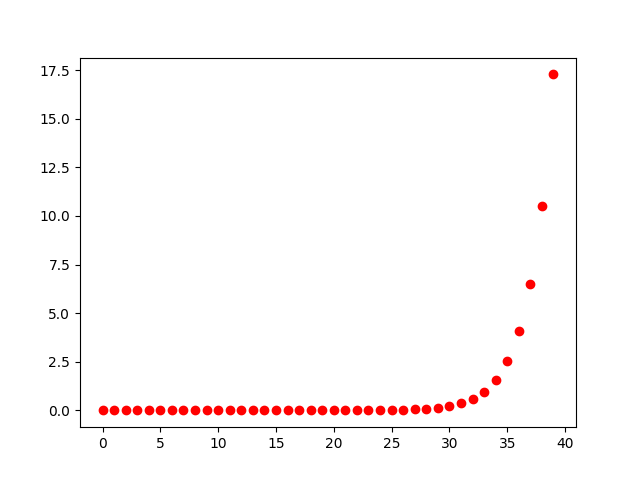a = b = 1
while b < 1000000000000:
print(b)
a, b = a+b, a$$fib(n) = \frac{1}{\sqrt{5}}(\frac{1+\sqrt{5}}{2})^n-\frac{1}{\sqrt{5}}(\frac{1-\sqrt{5}}{2})^n$$

12200160415121876738


def fib(n):
return (math.pow((1+math.sqrt(5))/2, n) - math.pow((1-math.sqrt(5))/2, n)) / math.sqrt(5)
print(int(fib(93)))
12200160415121913856mpfr_t phi;
unsigned long int precision, x=5;
uint64_t digits = DIGITS;

precision = ceill((digits+1)*logl(10)/logl(2));
mpfr_init2(phi, precision);
mpfr_sqrt_ui(phi, x, MPFR_RNDN);
mpfr_div_ui(phi, phi, 2.0, MPFR_RNDN);
mpfr_printf("%.10000Rf\n", phi);
mpfr_clear(phi);


fib(93) 到底有多少位數呢？ 我們可以用 2^n 作為 F(n) 的上界 ， 最後所需的位數至少就是 ceil(n*log(2))，相對應的我們運算中的浮點數精確度的要求，2^n 這個上界有點可恥粗糙但有用， 頂多會浪費點記憶體，最後除出來的小數點後面多幾個零而已，如果能套用更精確的上界當然更好。

mpfr 的函式庫設計精良，呼叫上非常直覺，這段程式碼其實就是寫公式解，應該滿好懂的， 程式碼在此

But，人生最厲害的就是這個 But，公式解真的有比較快嗎？

user system total
formulafib.c 57.39s 2.04s 1:01.09
fastfib.c 4.70s 0.20s 6.524

O(lg n) 的 fast-fibonacci 遠比 O(1) 的公式解來得快。